# Characterization of Recombination Effects in a Liquid Ionization Chamber Used for the Dosimetry of a Radiosurgical Accelerator

Engineering

### Summary

A growing number of radiation therapy devices offer the advantage of delivering the dose through very small beams to the tumor, allowing for increased conformity and higher doses per fraction. Many different detectors can be used for the dosimetry of these small fields. In the present study, the effect of ion recombination is investigated for a liquid ionization chamber using a stereotactic radiation therapy system.

Wagner, A., Crop, F., Lacornerie, T., Reynaert, N. Characterization of Recombination Effects in a Liquid Ionization Chamber Used for the Dosimetry of a Radiosurgical Accelerator. J. Vis. Exp. (87), e51296, doi:10.3791/51296 (2014).

### Abstract

Most modern radiation therapy devices allow the use of very small fields, either through beamlets in Intensity-Modulated Radiation Therapy (IMRT) or via stereotactic radiotherapy where positioning accuracy allows delivering very high doses per fraction in a small volume of the patient. Dosimetric measurements on medical accelerators are conventionally realized using air-filled ionization chambers. However, in small beams these are subject to nonnegligible perturbation effects. This study focuses on liquid ionization chambers, which offer advantages in terms of spatial resolution and low fluence perturbation. Ion recombination effects are investigated for the microLion detector (PTW) used with the Cyberknife system (Accuray). The method consists of performing a series of water tank measurements at different source-surface distances, and applying corrections to the liquid detector readings based on simultaneous gaseous detector measurements. This approach facilitates isolating the recombination effects arising from the high density of the liquid sensitive medium and obtaining correction factors to apply to the detector readings. The main difficulty resides in achieving a sufficient level of accuracy in the setup to be able to detect small changes in the chamber response.

### Introduction

Dosimetry in radiation therapy has been performed using gaseous ionization chambers for many years. These detectors perform well as far as “conventional” radiation therapy is concerned, i.e. large homogeneous (or slowly varying) fields are used. However many recent devices, such as the Cyberknife (Figure 1) system studied in this work, offer the possibility of using very small fields (down to 5 mm). Other devices produce highly modulated beam profiles such as in Intensity-Modulated Radiation Therapy (IMRT). Conventional air-filled detectors are not well-suited for these techniques1; in order to reach an acceptable spatial resolution the volume of the cavity would have to be reduced to a size where the chamber response would become too low. Diodes offer the advantage of smaller sensitive volumes and they are extensively used in small beam dosimetry. However they present other limitations such as scattering effects arising from their metallic shielding12,13.

In a liquid ionization chamber2 (LIC), the ionization density is much higher and thus the reduction of the sensitive volume is possible without compromising the detector response. Moreover the sensitive medium has a density close to that of water, reducing the fluence perturbations associated with an air cavity. These aspects make the LIC an interesting candidate for small beam dosimetry3-5.

There are nevertheless some issues to address before being able to perform routine dosimetric measurements with LICs. First, due to the higher ionization density the recombination effects are more important than in air-filled chambers6-8. Recombination can either be initial (an electron recombines with its mother ion) or general (two ions coming from different ionization events recombine). The latter is dependent on the dose rate incident on the detector; this means that relative dose measurements (i.e. dose profiles, percentage depth doses, output factors) can potentially undergo deviations due to the change in dose rate. Recombination is characterized by the general collection efficiency, defined as the ratio of the measured charge to the charge produced by the incident radiation and escaping initial recombination: f = QC/Q0. In gaseous detectors recombination effects are evaluated using the two-voltage method from the theory of Boag9,10, which cannot be applied in LICs11.

An alternative can be found in the use of the two-dose-rate method8, consisting of varying the dose rate to isolate the influence of general recombination and measure the general collection efficiency through the relation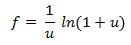where u is defined as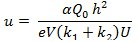with α being the recombination coefficient, Q0 the amount of charge that escapes initial recombination, h the electrode separation, e the elementary charge, V the sensitive volume of the chamber, k1 and k2 the mobilities of the positive and negative charges, and U the applied voltage. By measuring at different doses per pulse it is possible to obtain the parameter u and thus the collection efficiency, f. The dose per pulse is given by the relation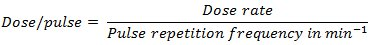All measurements are performed at the reference conditions of the Cyberknife (Source-Surface Distance SSD = 78.5 cm, 1.5 cm depth, 60 mm collimator). The use of a large collimator allows avoiding the volume effects associated with small beams. Given the dose rate is 800 MU/min and the repetition frequency is 150 Hz, this results in a dose of 0.89 mGy/pulse (at the reference conditions, 1 MU corresponds to a dose of 1 cGy). When the pulse repetition frequency is kept constant, the dose per pulse only depends on the dose rate in Gy/min, which is related to the SSD through the inverse-squared distance law: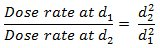for two SSDs d1 and d2.

### Protocol

1. Experimental Setup (Figure 2)

(Performed 1 hr prior to the first measurements to stabilize the detector temperature and the high voltage supply.)

1. Place the water tank under the treatment head, keeping in mind that the SSD will have to be increased up to 200 cm. Thus the tank should be positioned as low as achievable, depending on the ceiling height.
2. Align the water tank with the linac (its vertical sides must be parallel to the vertical sides of the head). The laser can be used to ensure the orientation is correct; this procedure is detailed in the Physics guide14 of the system.
3. Verify the vertical orientation of the linac by performing x and y profile measurements at two different depths, computing the beam declination and correcting using the axes of rotation of the head (see Physics guide).
4. Replace the collimator with the telemeter accessory, and use it to accurately position the head at 78.5 cm SSD. The tip of the accessory should barely touch the water surface.
5. Remove the telemeter and place the 60 mm collimator on the treatment head.
6. Position the LIC reference point at 1.5 cm depth in a vertical position, i.e. with the axis of the cylindrical cavity parallel to the beam direction. This results in a distance of 80 cm between the source and the detector. Use the laser to position the LIC at the center of the beam in the lateral direction.
7. Place a 0.125 cm3 air-filled ionization chamber (AIC) next to the LIC to be able to correct for attenuation, distance and scatter effects.
8. Connect the LIC and the high voltage supply to the electrometer and set the voltage to 800 V. Connect the AIC to another electrometer and set the voltage to 400 V. Then wait 1 hr for stabilization purposes.
9. To ensure the accuracy of the detector lateral positioning, perform profile measurements in both transverse directions and correct the zero of the LIC if necessary.
10. Make sure the repetition rate of the linac is fixed (nominal value = 150 Hz).

2. Measurements

1. First deliver a pre-irradiation dose of 3,000 monitor units (MU) in order to stabilize the LIC response. Then perform a zero of the electrometers.
2. To assess leakage current and stability, perform a series of charge acquisitions with the beam off for a duration equal to that of the measurements (7.5 sec for 100 MU). Compare the average value obtained to the values measured with the beam on. A typical leakage charge of less than 0.03% of the smallest value measured with the beam on can be considered negligible.
3. Place the treatment head at 58.5 cm SSD: use the remote controller in Cartesian mode and simply perform a 20 cm motion in the z-direction.
4. Leave the treatment room, close the door and program an irradiation of 100 MU at the operator console. Then start both electrometers, deliver the dose and note the charges measured by the LIC and the AIC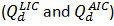.
5. Repeat the process 10 times to be able to assess statistical uncertainties.
6. After ten measurements, enter the room and move the treatment head to the next position (68.5 cm SSD). Then repeat steps 2.4 and 2.5.
7. When the head is moved further away from the tank the distance between measuring points can be increased as the charge varies following the inverse-squared distance law. Table 1 provides an example of a list of measuring points, along with the corresponding dose per pulse.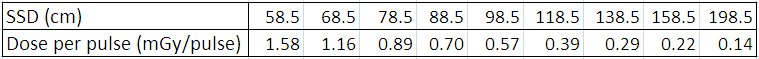Table 1. List of measuring points for the two-dose rate method (A and B) with the corresponding doses per pulse.

3. Analysis

Two methods can be used to analyze the data.

Method A

1. For every distance d, take the ratio of each measured LIC value with the corresponding AIC value obtained at the same distance,.
2. Plot the ratiosagainst the dose per pulse and use a linear fit to obtain the extrapolated ratio at zero dose per pulse, R0.
3. With the assumption that the collection efficiency is equal to 1 at 0 mGy/pulse, normalize all the ratios calculated in step 3.A.1 to the extrapolated value from the previous step in order to obtain values of f (i.e. scale every ratio by a factor such that k R0 = 1).
4. Plot the values of f against the values of the dose per pulse to represent the evolution of the collection efficiency. The error bars can be calculated by propagating the uncertainties on the LIC and AIC charges evaluated from the repeated measurements at each distance.

Method B

1. Take the ratios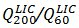of the LIC readings at 200 cm (198.5 cm SSD) and 60 cm (58.5 cm SSD), and of the AIC readings,.
2. Numerically solve the equation below for u200.3. Inject the value of u200 in the following relation to obtain the collection efficiency, f at 200 cm distance.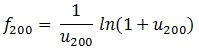4. The efficiency can be calculated using the ratios with other distances than 60 cm, as long as the charge ratio is higher than 3. This procedure allows testing the uncertainty on the calculated u and f values.
5. Calculate the parameter u for all measurement points, using the following relation (choosing the distances so that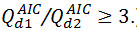)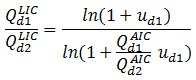6. Calculate all collection efficiencies, fd, from the relation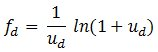7. Plot the values of f against the values of the dose per pulse to represent the evolution of the collection efficiency. The error bars can be calculated by propagating the uncertainties on the LIC and AIC charges evaluated from the repeated measurements at each distance.

### Representative Results

In Figure 3 the collection efficiency f obtained from method A is plotted against the dose per pulse, which ranges from 0 to 1.6 mGy/pulse where a 2% loss in signal can be seen. The points follow a linear behavior. The error bars show important uncertainties which seem inherent to the method and can be greatly reduced with the use of method B. It is also worth noting that in this method the AIC response is assumed to undergo no recombination effects, which is not necessarily entirely true. To verify this one can simply perform similar measurements using the AIC alone in a build-up cap (no water tank) and correct for the inverse square distance; small deviations can be observed and included in the uncertainties values.

Figure 4 shows the collection efficiency calculated from the second method (B). It proves more precise and has the advantage of providing absolute values of f. The deviations from the linear behavior are small and the loss in signal is slightly lower than with method A.

As a direct application of method B, factors can be calculated to correct for general recombination at a given dose per pulse, by simply taking the inverse of the collection efficiency, f. Then these factors can be applied to relative depth dose measurements. Figure 5 shows relative depth dose measured with a diode (not subject to recombination effects) and with the LIC before and after recombination correction. When the curves are normalized at a depth of 240 mm (where recombination effects vanish), they coincide, meaning that the corrections compensate for recombination effects in the buildup area (where the dose per pulse and thus the correction factors are the highest). This suggests that the calculated correction factors are accurate and can serve as a validation of the two-dose rate method.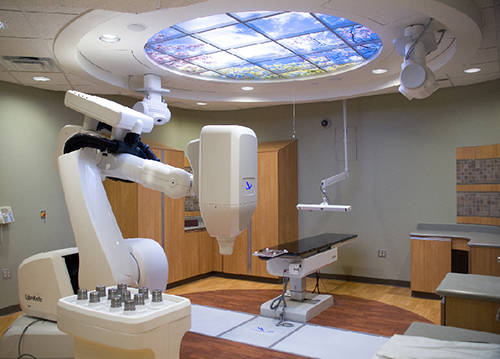Figure 1. Cyberknife sytem. A view of the Cyberknife system used for the measurements, with the accelerator head pointing downwards. The water tank can either be placed on the floor, or on the robotic couch visible at the back of the room, depending on the space available above the head.Figure 2. Experimental setup. The setup is represented here with the AIC and LIC placed next to each other inside the water tank (1.5 cm depth), at the center of the beam, which is directed downwards. The arrows indicate the accelerator head movement between each series of measurements, starting at 60 cm distance (58.5 cm SSD) and ending at 200 cm (198.5 cm SSD).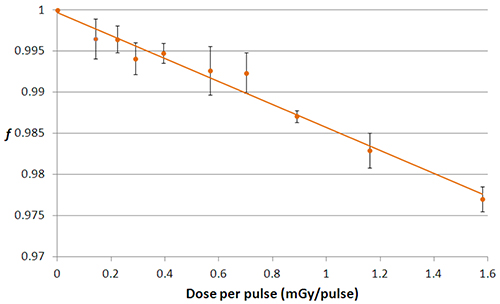Figure 3. General collection efficiency, method A. Graph of the evolution of the general collection efficiency, f, with respect to the dose per pulse (in mGy/pulse) obtained from method A.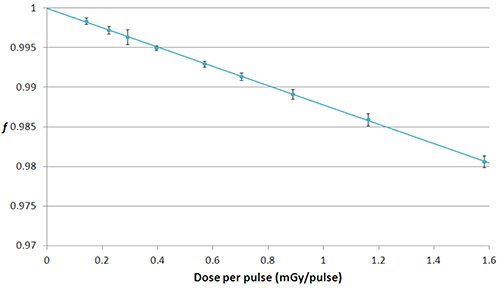Figure 4. General collection efficiency, method B. General collection efficiency plotted against the dose per pulse, following results from method B.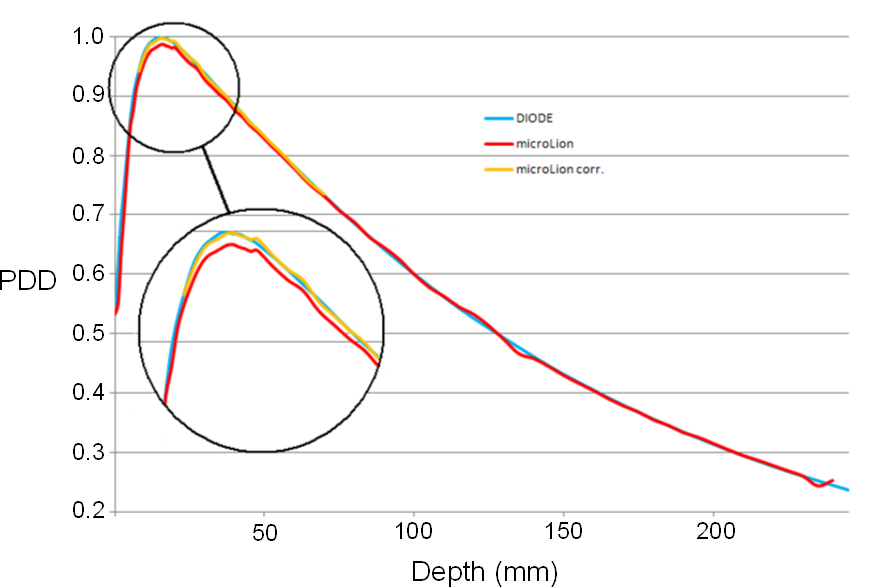Figure 5. Application to relative depth dose measurements. The relative depth dose obtained from the diode measurements is shown in blue. The results from the LIC measurements are represented by the red (uncorrected) and yellow (corrected) curves. Please click here to view a larger version of this figure.

### Discussion

The methods presented above allow evaluating the recombination effects in a LIC over a large range of dose rates (0.14 to 1.58 mGy/pulse). Method A is simple but is associated with more uncertainties than method B, which provides fairly accurate (and absolute) values of the collection efficiency, f. Recombination is responsible for approximately 2% loss in signal over the whole range investigated, but this range is larger than what is usually spanned during routine measurements. The largest error on an output factor is 0.35%, and it reaches 1% for a percentage depth dose measurement as was shown in the results section.

The critical element for conducting the protocol is the initial setup of the experiment, as all measurements are performed relative to the initial position of the treatment head. Thus one should be careful about the accurate measurement of the initial SSD to be able to relate the detector readings to each dose per pulse. This is also true for the placement of the detector in the water; care should be taken that the effective point of measurement (situated 1 mm behind the entrance window in the case of the microLion detector) is positioned at 1.5 cm below the surface. The 1 hr delay and the pre-irradiation dose are also essential in order to stabilize the 800 V supply and the temperature.

The repetition rate of the linac directly impacts the dose per pulse. At 800 MU/min and with a frequency of 150 Hz, the dose per pulse is 0.89 mGy/pulse. This frequency should be fixed for all measurements to make sure the distance is the only variable factor having an influence on the dose per pulse. The method can be used in the case of a continuous beam with some adaptations7. On other devices where the SSD cannot be varied by directly moving the treatment head, the repetition rate could be modified to introduce the dose per pulse variation. If this parameter is fixed as well, the SSD can still be modified by moving the LIC and the water surface in the tank, but the accuracy of this approach would likely be lower than the treatment head movement used in the present study.

The next step in the characterization of the LIC for its use in small field dosimetry is to investigate the other factors that induce perturbation of the response, such as the materials of the detector and the volume effect (i.e. the fact that the sensitive volume is not small compared to the dimensions of the beam). This is possible through the use of Monte Carlo simulations5. With those aspects taken into account, global correction factors can be applied to the LIC readings obtained in clinical routine measurements (output factors, percentage depth doses, dose profiles) in order to fully eliminate the perturbations.

After the complete characterization and correction of these perturbating effects, the LIC can be used as an additional detector for small beam dosimetry, allowing independent verification of profiles, percentage depth doses and output factors measured by other detectors. Its very high spatial resolution in the longitudinal direction would also be suited for the dosimetry of rectangular fields with only one small dimension (e.g. Tomotherapy.)

### Disclosures

The authors have nothing to disclose.

### Acknowledgments

The authors have no acknowledgements.

### Materials

 Name Company Catalog Number Comments MicroLion chamber PTW 31018 http://www.ptw.de/2263.html Unidos Webline dosimeter PTW http://www.ptw.de/unidos_webline_dosemeter_rt0.html HV supply PTW http://www.ptw.de/2265.html MP3 water scanning system PTW http://www.ptw.de/2032.html 0.125 cm3 SemiFlex chamber PTW 31010 http://www.ptw.de/semiflex_chambers0.html?&cId=6069 Cyberknife Accuray